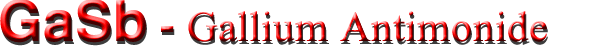Optical properties

 Index of refraction 3.8 Radiative recombination coefficient ~ 10-10 cm3s-1

Infrared refractive index
n = k1/2≈3.71·(1+8.25·10-5T)

Long-wave TO phonon energy hνTO = 27.78 meV (300 K).
Long-wave LO phonon energy hνLO = 28.89 meV (300 K).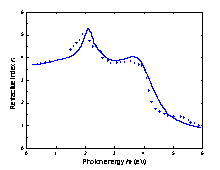Refractive index n versus photon energy, 300 K (Adachi (1989)).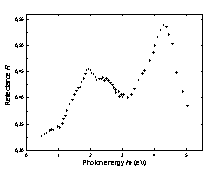Reflectivity versus photon energy, 300 K (Cardona (1961)).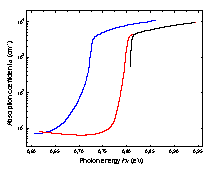Intrinsic absorption coefficient near the intrinsic absorption edge in pure p-type samples. T(K): 1. 300, 2. 77, 3. 4.2 (Becker et al. (1961)).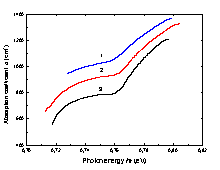Intrinsic absorption edge in p-type GaSb. Na = 3·1019 cm-3; T(K): 1. 215; 2. 140; 3. 77 (Iluridze et al. (1987)).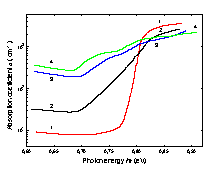Intrinsic absorption edge at 77 K for different doping levels, p-GaSb. Na(cm-3): 1. 2.9·1017; 2. 5·1018; 3. 1.8·1019; 4. 3·1019 (Iluridze et al. (1987)).
A ground state Rydberg energy RX1 = 2.8 meV.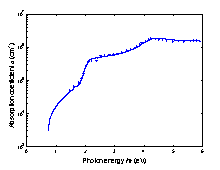The absorption coefficient versus photon energy, T=300 K (Adachi (1989)).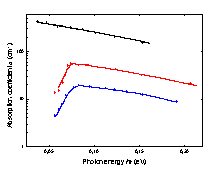The impurity absorption at low photon energies, T=80 K Undoped sample (p = 2.4·1017 cm-3 at 300 K) Te added (p = 7.5·1016 cm-3) Se added (p = 4.1·1016 cm-3) (Johnson and Fan (1965)).